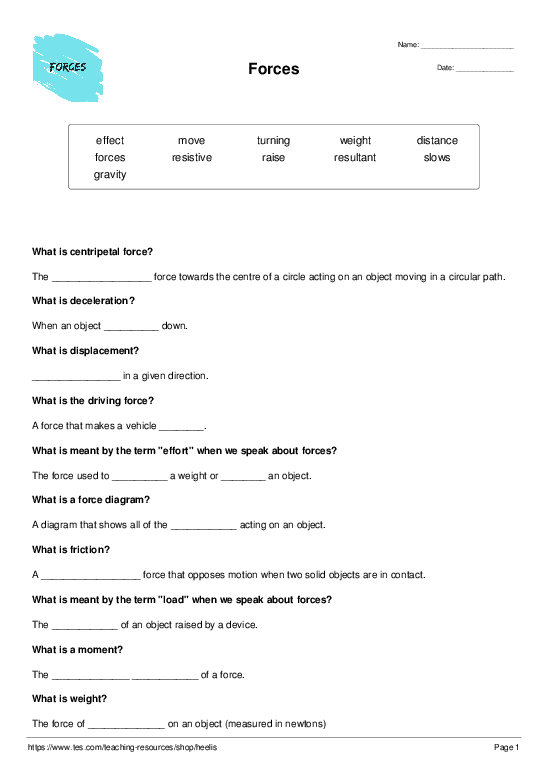# Forces

Cloze Test Worksheet

Kindly Shared By:United Kingdom

Date Shared: 23 September 2018

Worksheet Type:

Tags Describing Content or Audience:

Worksheet Instructions:

None provided.Target Language:

What is centripetal force? The resultant force towards the centre of a circle acting on an object moving in a circular path. What is deceleration? When an object slows down. What is displacement? Distance in a given direction. What is the driving force? A force that makes a vehicle move. What is meant by the term "effort" when we speak about forces? The force used to raise a weight or move an object. What is a force diagram? A diagram that shows all of the forces acting on an object. What is friction? A resistive force that opposes motion when two solid objects are in contact. What is meant by the term "load" when we speak about forces? The weight of an object raised by a device. What is a moment, and what is the equation to calculate a moment? The turning effect of a force. To calculate the turning effect use the equation - moment = force x perpendicular distance from the pivot to the line of action of the force What is weight? The force of gravity on an object (measured in newtons)

resultant slows Distance move raise move forces resistive weight turning effect gravity23 September 2018

#### heelisAuthorUnited Kingdom

Definitions Practice